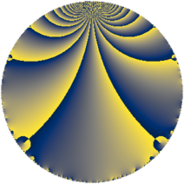Properties

 Label 105.3.rLevel $105$ Weight $3$ Character orbit 105.r Rep. character $\chi_{105}(19,\cdot)$ Character field $\Q(\zeta_{6})$ Dimension $32$ Newform subspaces $1$ Sturm bound $48$ Trace bound $0$

Related objects

Defining parameters

 Level: $$N$$ $$=$$ $$105 = 3 \cdot 5 \cdot 7$$ Weight: $$k$$ $$=$$ $$3$$ Character orbit: $$[\chi]$$ $$=$$ 105.r (of order $$6$$ and degree $$2$$) Character conductor: $$\operatorname{cond}(\chi)$$ $$=$$ $$35$$ Character field: $$\Q(\zeta_{6})$$ Newform subspaces: $$1$$ Sturm bound: $$48$$ Trace bound: $$0$$

Dimensions

The following table gives the dimensions of various subspaces of $$M_{3}(105, [\chi])$$.

Total New Old
Modular forms 72 32 40
Cusp forms 56 32 24
Eisenstein series 16 0 16

Trace form

 $$32 q + 32 q^{4} - 6 q^{5} - 48 q^{9} + O(q^{10})$$ $$32 q + 32 q^{4} - 6 q^{5} - 48 q^{9} + 78 q^{10} - 28 q^{11} + 60 q^{14} - 24 q^{15} - 40 q^{16} - 60 q^{19} + 12 q^{21} - 34 q^{25} - 96 q^{26} - 88 q^{29} + 84 q^{31} - 170 q^{35} - 192 q^{36} + 36 q^{39} + 330 q^{40} + 320 q^{44} + 18 q^{45} - 60 q^{46} + 356 q^{49} + 12 q^{51} - 468 q^{56} - 804 q^{59} - 198 q^{60} + 336 q^{61} - 400 q^{64} - 46 q^{65} - 108 q^{66} - 438 q^{70} + 344 q^{71} + 900 q^{74} + 144 q^{75} - 20 q^{79} + 1140 q^{80} - 144 q^{81} + 780 q^{84} + 304 q^{85} + 144 q^{86} + 24 q^{89} - 224 q^{91} - 924 q^{94} - 342 q^{95} + 900 q^{96} + 168 q^{99} + O(q^{100})$$

Decomposition of $$S_{3}^{\mathrm{new}}(105, [\chi])$$ into newform subspaces

Label Dim. $$A$$ Field CM Traces $q$-expansion
$a_{2}$ $a_{3}$ $a_{5}$ $a_{7}$
105.3.r.a $32$ $2.861$ None $$0$$ $$0$$ $$-6$$ $$0$$

Decomposition of $$S_{3}^{\mathrm{old}}(105, [\chi])$$ into lower level spaces

$$S_{3}^{\mathrm{old}}(105, [\chi]) \cong$$ $$S_{3}^{\mathrm{new}}(35, [\chi])$$$$^{\oplus 2}$$